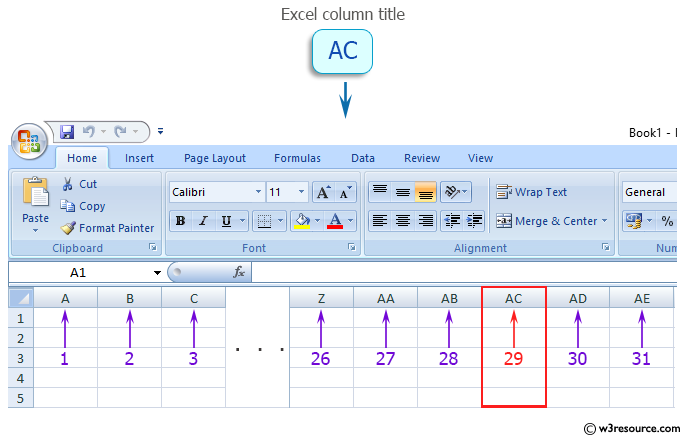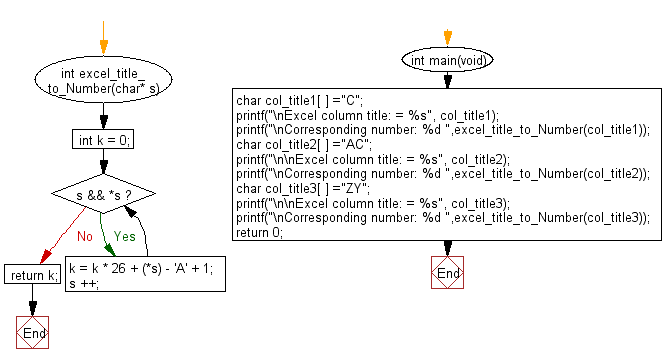﻿ C Program: Excel column number for a given title number

# C Exercises: Get the column number that corresponds to a column title as appear in an Excel sheet

## C Programming Mathematics: Exercise-9 with Solution

Write a C program to get the column number (integer value) that corresponds to a column title as it appears in an Excel sheet.

For example:
A -> 1
B -> 2
C -> 3
...
Z -> 26
AA -> 27
AB -> 28
...

Example:
Input:
col_title1[ ] ="C"
col_title2[ ] ="AC"
col_title3[ ] ="ZY"
Output:
Corresponding number: 3
Corresponding number: 29
Corresponding number: 701

Pictorial Presentation:Sample Solution:

C Code:

``````#include <stdio.h>

int excel_title_to_Number(char* s) {
int k = 0;
while (s && *s) {
k = k * 26 + (*s) - 'A' + 1;
s ++;
}
return k;
}

int main(void)
{
char col_title1[ ] ="C";
printf("\nExcel column title: = %s", col_title1);
printf("\nCorresponding number: %d ",excel_title_to_Number(col_title1));
char col_title2[ ] ="AC";
printf("\n\nExcel column title: = %s", col_title2);
printf("\nCorresponding number: %d ",excel_title_to_Number(col_title2));
char col_title3[ ] ="ZY";
printf("\n\nExcel column title: = %s", col_title3);
printf("\nCorresponding number: %d ",excel_title_to_Number(col_title3));
return 0;
}
```
```

Sample Output:

```Excel column title: = C
Corresponding number: 3

Excel column title: = AC
Corresponding number: 29

Excel column title: = ZY
Corresponding number: 701
```

Flowchart:C Programming Code Editor:

Improve this sample solution and post your code through Disqus.

What is the difficulty level of this exercise?

Test your Programming skills with w3resource's quiz.

﻿